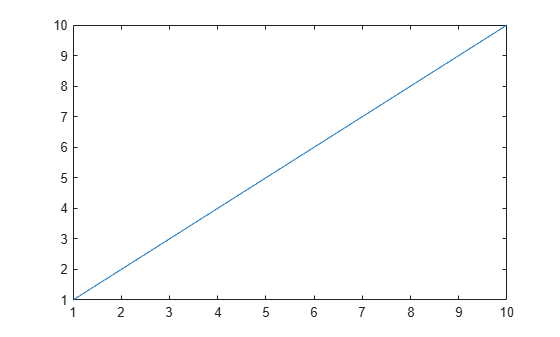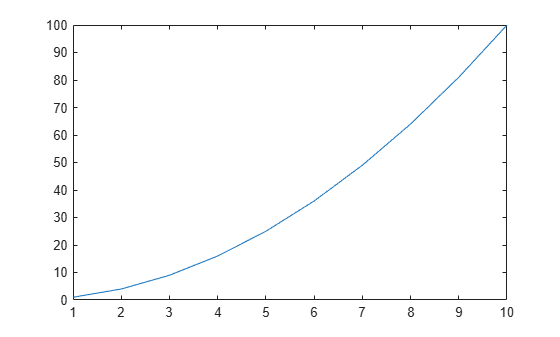# close

## 语法

``close``
``close(fig)``
``close all``
``close all hidden``
``close all force``
``status = close(___)``

## 说明

``close` 关闭当前图窗。调用 `close` 等效于调用 `close(gcf)`。`

``close(fig)` 关闭 `fig` 指定的图窗。`

``close all` 关闭其句柄可见的所有图窗。如果 `HandleVisibility` 属性设置为 `'callback'` 或 `'off'`，则隐藏图窗句柄。`

``close all hidden` 关闭所有图窗，包括具有隐藏句柄的图窗。 `

``close all force` 关闭所有图窗，包括已指定 `CloseRequestFcn` 回调以防止用户关闭图窗窗口的图窗。`

``status = close(___)` 返回上述任一语法的关闭操作的 `status`。如果一个或多个图窗关闭，函数将返回 `1`，否则返回 `0`。如果指定了 `status` 输出，则必须将字符向量形式的输入参数用括号括起来；例如，`status = close('all','hidden')`。`

## 示例

```f1 = figure; plot(1:10)``````f2 = figure; plot((1:10).^2)``````close(f1) f1```
```f1 = handle to deleted Figure ```

`close`

```f1 = figure; f2 = figure; f3 = figure; plot(1:10)````close([f1 f2])`

```figure(1) plot(1:10)``````figure(2) plot((1:10).^2)````close(2)`

```figure('Name','Measured Data'); plot(1:10)````close('Measured Data')`

```f1 = figure; plot(1:10)``````f2 = figure; plot((1:10).^2)````status = close(f1)`
```status = 1 ```

```f1 = figure; plot(1:10)``````f2 = figure; plot((1:10).^2)``````f3 = figure; plot(1./(1:10))````close all`

```f1 = figure; plot(1:10)``````f2 = figure; plot((1:10).^2)``````f3 = figure('HandleVisibility','off'); plot(1./(1:10))````close all hidden`

`CloseRequestFcn` 属性使您能够指定关闭请求回调，每当用户尝试关闭图窗窗口时都会执行该回调。例如，您可以显示一个对话框，要求确认或取消关闭操作，或者阻止用户关闭含有 UI 的图窗。

```f1 = figure('CloseRequestFcn',''); plot(1:10)``````f2 = figure; plot((1:10).^2)````close all force`

## 输入参数

• 如果 `fig` 是图窗编号，则 MATLAB® 会搜索 `Number` 属性等于 `fig` 的现有图窗。默认情况下，`Number` 属性值显示在图窗的标题中。

• 如果 `fig` 是图窗名称，则 MATLAB 会搜索 `Name` 属性等于 `fig` 的现有图窗。

## 提示

• 要无条件删除所有图窗，请使用以下语句：

```set(groot,'ShowHiddenHandles','on') c = get(groot,'Children'); delete(c)```

• 实现 `CloseRequestFcn` 回调时，不要调用 `close`。在回调的主体中调用 `close` 会产生导致 MATLAB 警告的递归。应使用 `delete` 函数实现回调。`delete` 会删除图窗，而不执行 `CloseRequestFcn` 回调。

• 如果您在未指定 `CloseRequestFcn` 属性的情况下对图窗调用 `close``closereq` 属性的默认值会无条件地删除该图窗并关闭其窗口。要防止在调用 `close` 时删除，请实现 `CloseRequestFcn` 回调。

## 算法

`close` 函数使用以下语句计算指定图窗 `f``CloseRequestFcn` 属性：

`eval(get(f,'CloseRequestFcn'))`

`CloseRequestFcn` 使您能够在调用 `close` 后延迟或中止图窗的关闭。例如，可以显示一个对话框以确实用户是否真正希望关闭图窗或在关闭前保存并清理。

`CloseRequestFcn` 的默认值 `closereq` 使用 `delete(get(groot,'CurrentFigure'))` 关闭当前图窗。如果您指定了图窗句柄数组，`close` 会对每个图窗执行由 `CloseRequestFcn` 指定的回调。